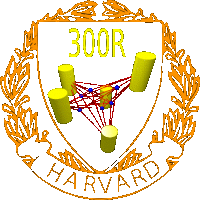Omni 3D project
300R: Mathematics of 3D image synthesis
Papers
Office: SciCtr 434
 A camera Q is a map from a manifold N onto itself such that Q2=Q and such that the image of Q is diffeomorphic to a fixed lower dimensional manifold S called retinal surface. Given a space of cameras modeled by a manifold M and retinal manifold S, the structure of motion problem asks to reconstruct points P1,...,Pm and cameras Q1,...Qn from the image data Qj(Pi). The structure from motion map F: Nn x Mm to Sn m is in general nonlinear. The goal is to find a locally unique reconstruction modulo a global symmetry group G and find conditions under which a globally unique reconstruction is possible. In case of a locally unique reconstruction, we want fast and explicit inversion formulas. In this summer 2007 project, we focussed on (1) the general structure from motion problem, (2) the problem for reconstruction for orthographic cameras and (3) the reconstruction problem for omnidirectional cameras. The course work is contained in the following three preprints:

 Paper Abstract A structure from motion inequality We set the general framework and state an inequality, which answers the question how many cameras and points are needed to invert the structure from motion map F. The paper includes the above mathematical definition of the structure from motion problem which is general enough to cover situations, where points are moving while the camera takes pictures. The point manifold N could be a space of jets of functions Pi(t). Inverting the structure from motion map means recovering the paths of the points and the camera locations from the camera pictures. We illustrate the inequality for various camera types. On Ullmans theorem in computer vision We provide explicit locally unique reconstruction of the structure from motion map F in the case of three orthographic cameras and three points both in 2 and 3 dimensions. While Ullmans theorem shows that the structure from motion map F is 2:1 for 3 cameras and 4 points, the reconstruction is 64:1 for 3 cameras and 3 points but in the later case, the map F has an image which has the same dimension. In Ullmans case, the image of F is a codimension 3 manifold. We also demonstrate that the map F is not surjective. Its boundary is the image of the set det(F)=0. Space and camera path reconstruction for omnidirectional vision For oriented omnidirectional cameras, the structure from motion map can be reduced to linear problems. We give a complete answer for the question when a unique reconstructions is possible without ambiguities. For example, for 3 points and 3 cameras in the plane, a unique reconstruction of points and cameras is possible if the 3 cameras are not collinear, the 3 points are not collinear and the 6 points are not contained in the union of two lines. This is sharp and relaxing any of these conditions leads to counter examples. Proving these facts involves elementary geometry like Desargues theorem. We do the reconstruction for arbitrarily many cameras and arbitrarily many points using least square solutions and show that it is robust. The goal will be to take a movie with many frames, observe many points and do a reconstruction both of the camera path and the scene. We have programs to do the reconstruction with synthetic data for arbitrary many points and cameras.